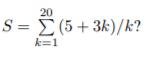Thread: Finding the value of the 5th term of a sum

1. Finding the value of the 5th term of a sum

The question asks to find the value of the 5th term of the sum:I just did it by hand by inputting k=1, then k=2 and so on till k=5 and I summed them all up. I got 19.41. However, the answer key says 4. Can anyone tell me how to do this question. Also, is there some general formula for these types of questions, instead of doing it the way I did.

2. Re: Finding the value of the 5th term of a sum

From the given sum, we see that the $\displaystyle k$th term of the sum is given by:

$\displaystyle \frac{3k+5}{k}$

And so the 5th term is:

$\displaystyle \frac{3(5)+5}{5}=\frac{4\cdot5}{5}=4$

3. Re: Finding the value of the 5th term of a sum

Latinized, you were asked for the fifth term. What you calculated was the sum of the first 5 terms.